##### How to Subtract Days From a Date in PostgreSQL

Subtracting days from a date is a common task that you may need to perform while calculating the expiry dates, finding the difference between two dates, or simply adjusting a date to a past or future date. Thankfully, Postgres offers some in-built functions and operators that make it easy to subtract days from a date.

This Postgres blog will teach you how to use the Postgres INTERVAL, DATE_PART() function, and the minus-” operator to subtract days from a date. The content of this blog will be organized as follows:

• Subtracting Days From a Date Using a MINUS Operator
• Subtracting Days From a Date Using INTERVAL
• Subtracting Days From a Date Using DATE_PART() Function

So, let's get started!

Subtracting Days From a Date Via a MINUS Operator

To subtract a single or multiple days from a date, use the minus "-" operator as follows:

`DATE 'dateField' - days;`

In place of days, specify the number of days to be subtracted.

Example 1: How to Get the Current Date in Postgres?

In Postgres, the CURRENT_DATE and NOW() functions are used to get the current date. In the following example, we will use the CURRENT_DATE function to find today’s date:

`SELECT CURRENT_DATE;`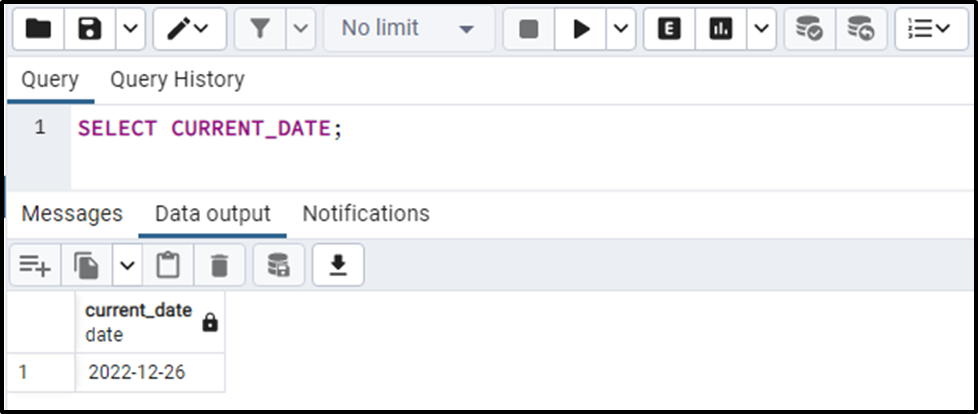The output snippet shows that today’s date is 26th December 2022.

Example 2: Subtract One Day From the Current Date

The below statement shows how to subtract a day from today’s date:

`SELECT CURRENT_DATE - 1;`

In the above snippet, the CURRENT_DATE is used to get today’s date, while “1” represents the number of days to be subtracted from the current date: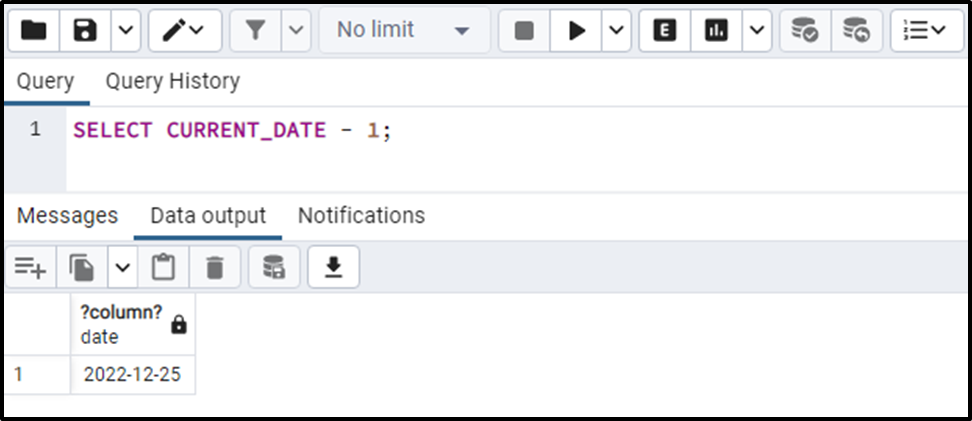The output shows that the MINUS operator subtracted one day from the current date.

Example 3: Subtract Multiple Days From Current Date?

To subtract multiple days from the current date, you need to specify the CURRENT_DATE followed by the minus operator and then the number of days to be subtracted :

`SELECT CURRENT_DATE - 12;`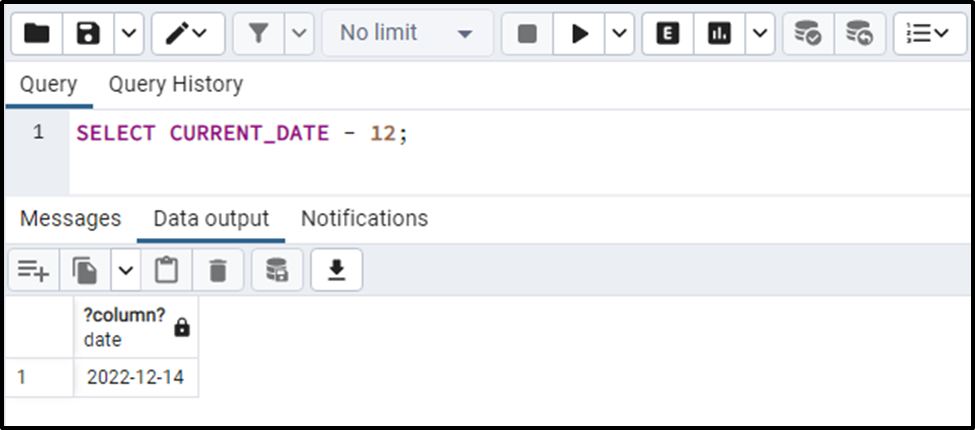The output verifies the working of the minus operator.

Example 4: Subtract Days From a Specific Date?

Let’s learn how to subtract days from a specific date field using the minus operator:

`SELECT DATE '2019-11-14' - 18;`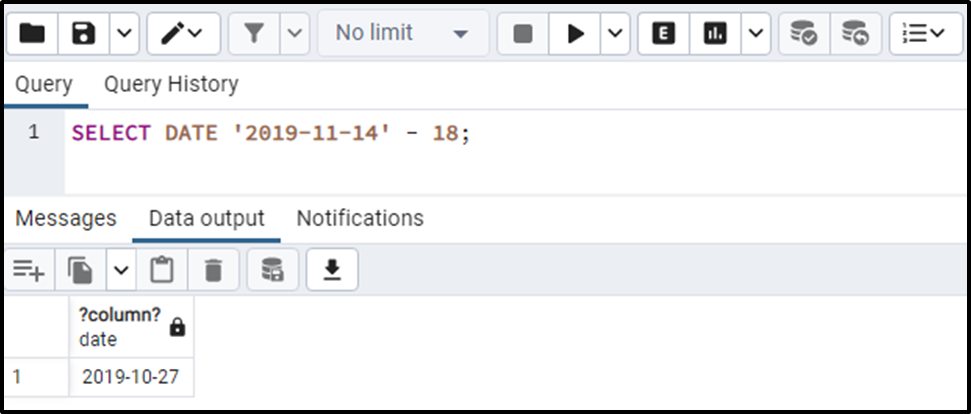The output snippet authenticates the working of the minus operator.

Subtracting Days From a Date Via INTERVAL

Another very convenient way to subtract days from the date is INTERVAL, as shown in the following snippet:

`DATE 'dateField' - INTERVAL 'no_of_days';`

In place of days, specify the number of no_of_days to be subtracted.

Example 1: How to Subtract Specific Days From a Date Using Interval?

Let’s learn how to use interval for subtracting days from a date in Postgres:

`SELECT DATE '2022-10-22' - INTERVAL '8 days';`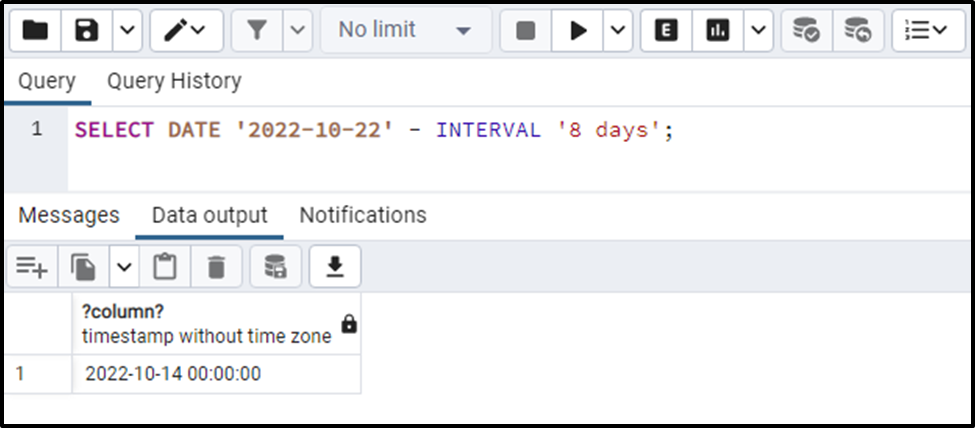The output snippet clarifies that the specified days have been subtracted from the given date.

Example 2: Specify Days Equivalent Hours to Subtract Days From a Date Using Interval

In the following snippet, we will subtract two days from a specific date field by specifying the hours instead of days:

`SELECT DATE '2022-10-22' - INTERVAL '48 hours';`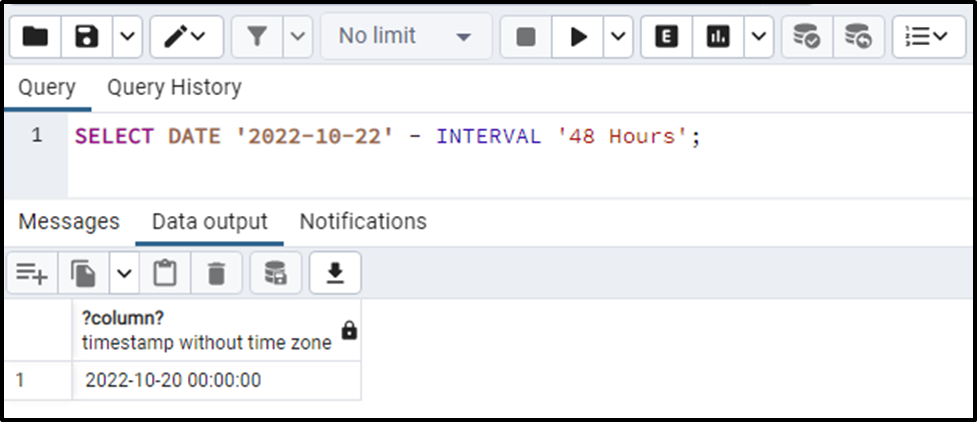The output snippet clarifies that the two days have been subtracted from the given date.

Subtracting Days From a Date Via DATE_PART() Function

Postgres offers a built-in DATE_PART() function that can be used to subtract the days from a month. To do so, use the below-provided syntax:

`DATE_PART('day', 'dateField' - no_of_days);`

Specify the days to be subtracted in place of “no_of_days”.

Example 1: How to Subtract Days From Current Date Using DATE_PART() Function?

In the following example, we will subtract seven days from the current date:

`SELECT DATE_PART('day', CURRENT_DATE - 7);`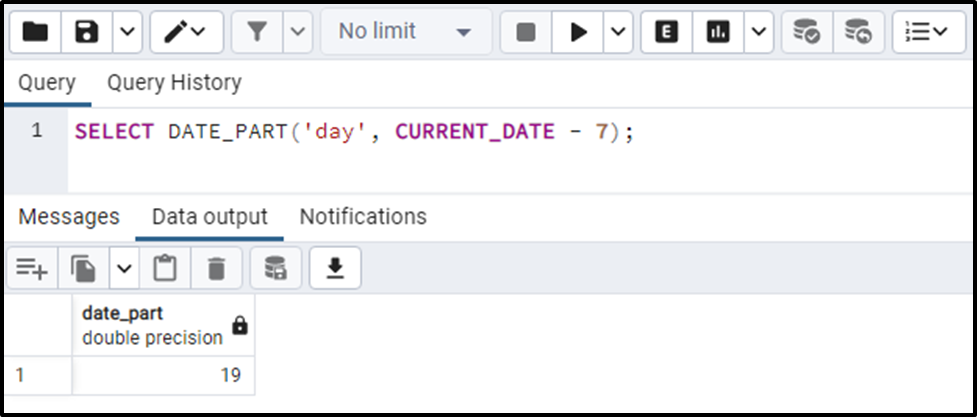The output snippet proves the appropriateness of the DATE_PART() function.

Example 2: How to Subtract Days From a Specific Date Using DATE_PART() Function?
The below piece of code will subtract twelve days from the given date field via the DATE_PART() function:

`SELECT DATE_PART('day', DATE '2022-12-12' - 7);`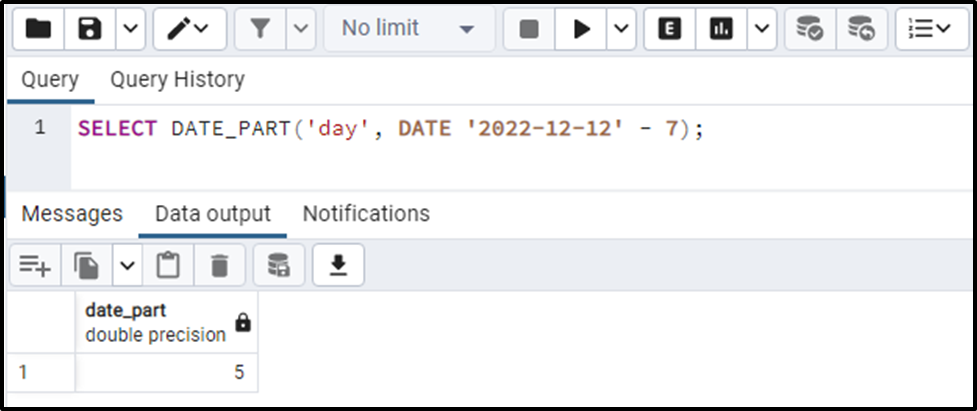The output shows that the DATE_PART() function subtracted the specified days from the given date field.

Conclusion

In PostgreSQL, the DATE_PART() function, INTERVAL, and the minus “-” operator is used to subtract a single or multiple days from a particular date. The MINUS operator and INTERVAL are the most convenient and widely used approaches for subtracting the days from a date. This Postgres blog explained various methods for subtracting days from a date in Postgres.